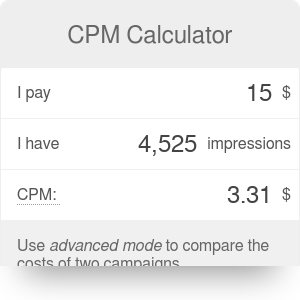# CPM Calculator

By Mateusz Mucha
CPM calculator helps you with cost and volume of advertising traffic.

CPM calculator helps compute a basic task for online marketers and publishers. Knowing how to calculate CPM comes into play when advertising budgets are considered. CPM is shorthand for cost per mile or cost per thousand and is a common measure of volume in advertising. This calculator works either way, so it may be used to calculate either the cost, CPM or number of impressions. If you'd like to combine it with cost per click, use our CPC and CPM calculator. Online marketers also love the online conversion calculator (check it out, it's a powerful tool!).

## How to calculate CPM

The formula for CPM is as simple as the concept behind it. Since CPM is cost per thousand impressions, then you simply divide the cost by the number of impressions divided by a thousand. So the CPM formula is `CPM = 1000 * cost / impressions`. What may interest you more is one of the reversed equations:

• for cost (how much you'll have to pay): `cost = CPM * impressions / 1000`
• for impressions (how many impressions you're going to get, given your budget): `impressions = 1000 * cost / CPM`

Mateusz Mucha

## Get the widget!

CPM Calculator can be embedded on your website to enrich the content you wrote and make it easier for your visitors to understand your message.

It is free, awesome and will keep people coming back!